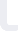Matrix Algebra Udemy

# Matrix Algebra هذه الدورة تدرس باللغة الإنجليزية

## Udemy

السعر: 25 USD

### تفاصيل الدورة

This course would help in developing understanding on Matrix Algebra. It starts with a lecture on Introduction to matrices to build(brush) up fundamentals and then progresses towards discussing intermediate levels topics including Algebra of matrix, Transpose and Determinants of a matrix, Adjoint and Inverse of matrix.

Throughout the course, emphasis is on learning Matrix Algebra using practice problems. Practical applications of theoretical concepts is of paramount importance too. There is a section dedicated in applying Matrix Algebra constructs for solving system of linear equations.

Curriculum for the course is organized into five different sections containing 12 video lectures and around 3 hours of content. Every section is coupled with sample solved examples and practice problems in the form of a Quiz.

This course is continuously monitored and I shall be more than happy in assisting students on Matrix Algebra queries.

Last but certainly not the least - Feedback is much appreciated!!

"Happy Learning" !!

تحديث بتاريخ 27 December, 2017
دورات يمكنك الالتحاق بها على الفور... Advanced Algebra: Strategies for Success Udemy Learn Algebra, from Beginner to Advanced equations Udemy Algebra I (Beginning Algebra) Udemy